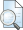## ANALISIS VALUE FOR MONEY UNTUK MENGUKUR KINERJA KEUANGAN PEMERINTAH KOTA MALANG

Hadi, Arizona (2009) ANALISIS VALUE FOR MONEY UNTUK MENGUKUR KINERJA KEUANGAN PEMERINTAH KOTA MALANG. Other thesis, University of Muhammadiyah Malang.Preview
Text
ANALISIS_VALUE_FOR_MONEY_UNTUK_MENGUKUR_KINERJA_KEUANGAN_PEMERINTAH_KOTA_MALANG.pdf - Published Version

## Abstract

Target of this research is to measure the performance of finance of Malang City Government through the method of value for money. Analysis technique that isused to measure the performance of finance of Malang City Government through the method of value for money that is by counting ratio effectivity, efficiency ratio, and economic ratio. to determine that performance have effective is if obtained by a value less than 100% ( x < 100%) meaning effective, if obtained by value of is equal to 100% ( x = 100%) meaning proportional efektifit, if obtained by a value more than 100% ( x > 100) meaning is not effective. Later;Then to determine that performance have efficient is if result of is percentage of under standard ( budget) mean efficient, if result of is percentage of residing in to the standard ( budget), meaning inefficient, If result of is percentage of is equal to standard ( budget), meaning proportional efficient. Meanwhile, to determine that performance have economic is if obtained by a value less than 100% ( x < 100%) meaning economic, if obtained by value of is equal to 100% ( x = 100%) meaning proportional economics, if obtained by a value more than 100% ( x > 100%) meaning is not economic. Pursuant to calculation analyse the value for money, average value of during three year for the ratio of effectivity is 96,74 % meaning Malang City Government have effective in managing its finance because ratio value less than 100 %. Later;Then mean for the ratio of efficiency is 96,74 % meaning efficient because ratio value less than the percentage of budget that is 102,21 %. But average value for the ratio of economic is 98,18% meaning Malang City Government still less economic in managing it’s finance because ratio value less than 100 %. So that during period 2005, 2006 , and 2007 inferential that Governmental finance performance of Malang City show the existence of good performance.

Item Type: Thesis (Other) H Social Sciences > HB Economic Theory Faculty of Economics and Business > Department of Accounting (62201) Anwar Jasin 28 Apr 2012 03:46 28 Apr 2012 03:46 http://eprints.umm.ac.id/id/eprint/3274

### Actions (login required)View Item